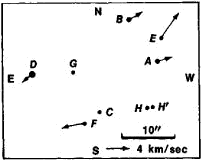# Trapezium

(redirected from trapeziums)
Also found in: Dictionary, Thesaurus, Medical.

## trapezium

1. Chiefly Brit a quadrilateral having two parallel sides of unequal length
2. Now chiefly US and Canadian a quadrilateral having neither pair of sides parallel
3. a small bone of the wrist near the base of the thumb

## Trapezium

(tră-pee -zee-ŭm) A very young open cluster of stars, protostars, gas, and dust that lies in the Orion nebula and is part of the Orion association. There are four prominent young main-sequence stars, which form the multiple star θ1 Orionis and are of spectral class O6, B1, B1, and B3. These stars excite and ionize the nebula. They form a trapezium and are visible with a small telescope.

## Trapezium

the multiple star θ1 Orionis, located in the middle part of the Great Nebula of Orion. The star has nine known components, the four brightest of which (A, B, C, and D in Figure 1) form the vertices of a trapezium.Figure 1. The Trapezium; the arrows at six of the stars indicate their proper motions over 1,000 years and the tangential velocity components on the scale indicated in the figure

In 1949, V. A. Ambartsumian discovered a number of similar stellar systems, in which the distances between components are of the same order of magnitude. Such systems came to be called Trapezium-type multiple stars. The periodic orbital motions observed in ordinary multiple stars are unlikely in Trapezium systems; consequently, such systems should be regarded as unstable and in the process of breaking up. According to Ambartsumian’s determinations, Trapezium systems break up in a few million years. It follows that such systems observed at the present time cannot be older than a few million years and are therefore young formations. A considerable number of Trapezium-type multiple stars are found in stellar associations.

## trapezium

[trə′pē·zē·əm]
(mathematics)
A quadrilateral where no sides are parallel.

## Trapezium

[trə′pē·zē·əm]
(astronomy)
Four very hot stars that appear to the eye as a single star in the Great Nebula of Orion; the star symbol is M42.
References in periodicals archive ?
The Steiner Theorem for Trapezium (Jakob Steiner, 1796-1863)
For every trapezium ABCD, the following four points--the midpoints of each base, the point where the diagonals cross, and the point of meeting of the continuation of the sides--are on the same line.
The students noted that Steiner's proof was comparable to the solution of their problem (the proof of which is given below) and thus were stimulated to continue researching the use of the Steiner theorem for the trapezium, which ultimately led to an interest in general geometric constructions according to the rules of ancient Greek mathematics, and building geometric figures using a straightedge only.
Steiner's proof of the trapezium theorem: To prove the theorem, it will be proven that the line EF intersects the bases of the trapezium at the points M and N (Figure 3).
From the theorem "the point of intersection of the diagonals of a trapezium bisects the segment parallel to the base whose ends are on the sides of the trapezium and which passes through the point of intersection" (can be proven using Thales' theorem), it is concluded that KE = EL, that is, EF is a median to the side KL in triangle [DELTA]FKL.
At the end of the article is another example for a geometrical problem that can be solved simply using Steiner's theorem for the trapezium, as well as an application of the presented constructions.
If U, V are arbitrary free finite points then SFIP can transform a universal parallelogram into a trapezium (by swapping the points [V.
infinity]] with the free finite points U, V, W will transform the parallelepiped into a prism, a truncated pyramid, a pyramid with a base, a parallelogram, trapezium, quadrilateral or triangle.
Applying the SFIP function the parallelepiped transforms instantly into a prism, a truncated pyramid, or a pyramid; with a parallelogram, trapezium, quadrilateral or triangular base, with a special positioning of a chosen vertex (Figures 6.
infinity]] with U the students obtain the trapezium ABCD on the computer screen straight away.
They transformed the hat, the body from a trapezium into a quadrilateral or a parallelogram, and changed the shapes of the head, eyes, hands, and feet.

Site: Follow: Share:
Open / Close